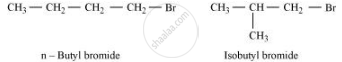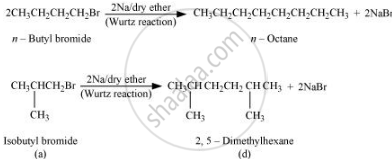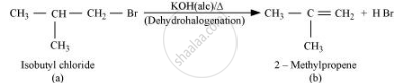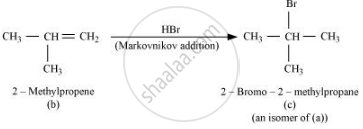Share

Primary alkyl halide C4H9Br (a) reacted with alcoholic KOH to give compound (b).Compound (b) is reacted with HBr to give (c) which is an isomer of (a). - CBSE (Science) Class 12 - Chemistry

ConceptMethods of Preparation of Haloalkanes and Haloarenes

Question

Primary alkyl halide C4H9Br (a) reacted with alcoholic KOH to give compound (b).Compound (b) is reacted with HBr to give (c) which is an isomer of (a). When (a) is reacted with sodium metal it gives compound (d), C8H18 which is different from the compound formed when n-butyl bromide is reacted with sodium. Give the structural formula of (a) and write the equations for all the reactions

Solution

There are two primary alkyl halides having the formula, C4H9Br. They are n − bulyl bromide and isobutyl bromide.Therefore, compound (a) is either n−butyl bromide or isobutyl bromide.

Now, compound (a) reacts with Na metal to give compound (b) of molecular formula, C8H18, which is different from the compound formed when n−butyl bromide reacts with Na metal. Hence, compound (a) must be isobutyl bromide.Thus, compound (d) is 2, 5−dimethylhexane.

It is given that compound (a) reacts with alcoholic KOH to give compound (b). Hence, compound (b) is 2−methylpropene.Also, compound (b) reacts with HBr to give compound (c) which is an isomer of (a). Hence, compound (c) is 2−bromo−2−methylpropane.Is there an error in this question or solution?

APPEARS IN

NCERT Solution for Chemistry Textbook for Class 12 (2018 to Current)
Chapter 10: Haloalkanes and Haloarenes
Q: 21 | Page no. 312

Video TutorialsVIEW ALL 

Solution Primary alkyl halide C4H9Br (a) reacted with alcoholic KOH to give compound (b).Compound (b) is reacted with HBr to give (c) which is an isomer of (a). Concept: Methods of Preparation of Haloalkanes and Haloarenes.
S